# KSEEB Solutions for Class 8 Maths Chapter 7 Rational Numbers Ex 7.4

Students can Download Maths Chapter 7 Rational Numbers Ex 7.4 Questions and Answers, Notes Pdf, KSEEB Solutions for Class 8 Maths helps you to revise the complete Karnataka State Board Syllabus and score more marks in your examinations.

## Karnataka Board Class 8 Maths Chapter 7 Rational Numbers Ex 7.4

1. Represent the following rational numbers on the number line.

Question (i)
$$\frac{-8}{5}=-2 \frac{2}{3}$$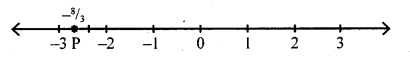Question (ii)
$$\frac { 3 }{ 8 }$$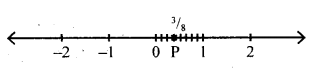Question (iii)
$$\frac { 2 }{ 7 }$$Question (iv)
$$\frac{12}{5}=2 \frac{2}{5}$$Question (v)
$$\frac{45}{13}=3 \frac{6}{13}$$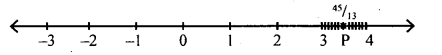Question 2.
Write the following rational numbers in ascending order.
$$\frac{3}{4}, \frac{7}{12}, \frac{15}{11}, \frac{22}{19}, \frac{101}{100}, \frac{-4}{5}, \frac{-102}{81}, \frac{-13}{7}$$
LCM of the denominators in 35550900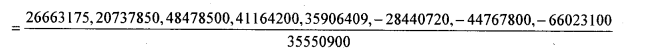= 66023100 < 44767800 < 2840720 < 20737850 < 26663175 < 35906409 < 41164200 < 48478500
∴$$\frac{-13}{7}<\frac{-102}{81}<\frac{-4}{5}<\frac{7}{12}<\frac{3}{4}<\frac{101}{100}<\frac{22}{19}<\frac{15}{11}$$

Question 3.
Write 5 rational number between $$\frac { 2 }{ 5 }$$ and $$\frac { 3 }{ 5 }$$ having same denominator.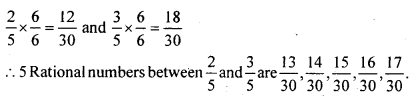Question 4.
How many positive rational numbers less than 1 are there such that the sum of the denominator and numerator does not exceed 10?
$$\frac{1}{2}, \frac{1}{3}, \frac{1}{4}, \frac{1}{5}, \frac{1}{6}, \frac{1}{7}, \frac{1}{9}, \frac{1}{3}, \frac{2}{3}, \frac{2}{3}, \frac{2}{5}, \frac{2}{4}, \frac{3}{4}, \frac{3}{5}, \frac{3}{7}, \frac{4}{5}$$
are lesser than 1. There are 15 rational numbers.

Question 5.
Suppose $$\frac { m }{ n }$$ and $$\frac { p }{ q }$$ are two positive numbers. Where does $$\frac{m+p}{n+q}$$ lie, with respect to $$\frac { m }{ n }$$ and $$\frac { p }{ q }$$ .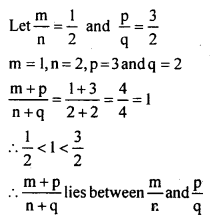Rational numbers with denominator 80 and numerator from 1 to 79 $$\left(\text { like } \frac{1}{80}, \frac{2}{80},-\cdots-\frac{79}{80}\right)$$